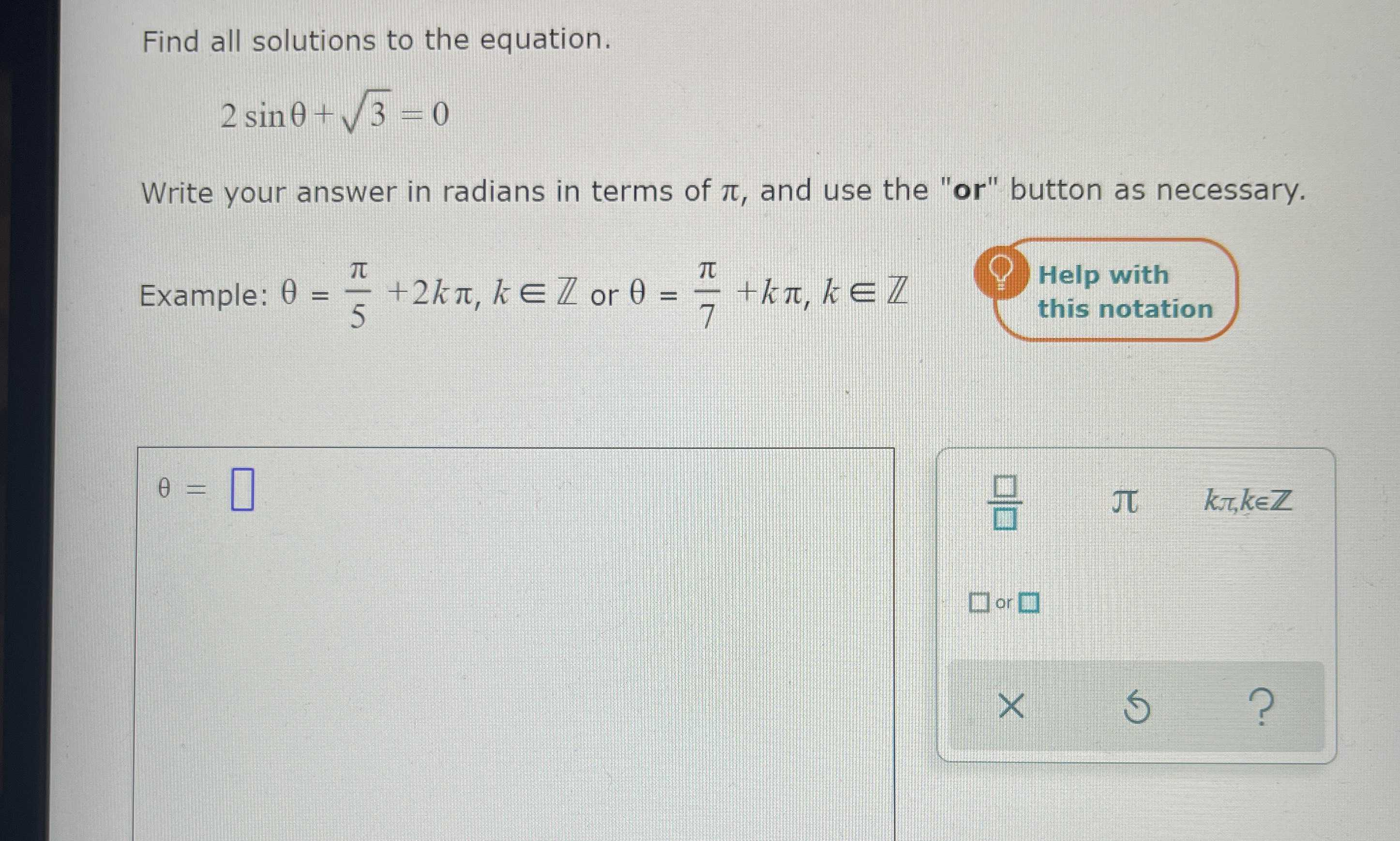### Still have math questions?

Trigonometry
QuestionFind all solutions to the equation.

$$2 \sin \theta + \sqrt { 3 } = 0$$

Write your answer in radians in terms of $$\pi$$ , and use the "or" button as necessary. Example: $$\theta = \frac { \pi } { 5 } + 2 k \pi , k \in Z$$ or $$\theta = \frac { \pi } { 7 } + k \pi , k \in Z$$ this notation

$$\theta = \square$$

$$- \frac{\pi }{3}$$+2k$$\pi$$, k$$\in$$Z or -$$\frac{2\pi }{3}$$+2k$$\pi$$, k$$\in$$